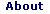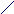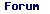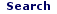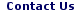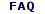Mon - 01 Jun 2020

WELCOME TO RFCABLES.ORG

## dBm to mW Calculator

### MilliWatt (mW) Definition

A milliwatt (mW) is one thousandth of a watt (W), or there are 1000 milliwatts in a watt. The watt is an SI derived unit of power. One watt is one joule of energy per second.

The formula to calculate mW from dBm is: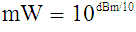dBm Definition

Enter value to convert from dBm to milli-watt (mW) below.

 Enter dBm =In milli watts:(Value Rounded to 4 digits after decimal)

mW to dBm Calculator

dBm to milli-Watt Table

milli-Watt to dBm Table